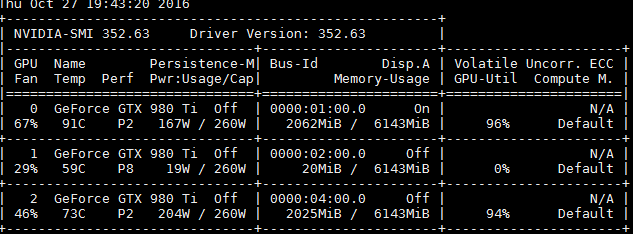1、运行多程序，但返回显存不足

1.1：theano后台限制显存：每个用户在自己home根目录建立.theanorc文件（注意这个文件默认不存在，且文件名前面有个点），配置参数例子如下（参考：config – Theano Configuration）：

``````[global]
floatX = float32
device = gpu0
optimizer_including = cudnn

[lib]
cnmem = 0.3

[cuda]
root = /usr/local/cuda/
``````

1.2：tensorflow后台限制显存：

tensorflow如果如果单纯用tensorflow的话可以用代码控制（参见：tensorflow.org/versions）：

``````config = tf.ConfigProto()
config.gpu_options.per_process_gpu_memory_fraction = 0.4
session = tf.Session(config=config, ...)
``````

``````import tensorflow as tf
from keras.backend.tensorflow_backend import set_session
config = tf.ConfigProto()
config.gpu_options.per_process_gpu_memory_fraction = 0.3
set_session(tf.Session(config=config))
``````

2、只运行了一个程序，运行返回显存不足

fit_generator方法定义

``````def fit_generator(self, generator, samples_per_epoch, nb_epoch,
verbose=1, callbacks=[],
validation_data=None, nb_val_samples=None,
class_weight=None, max_q_size=10, **kwargs):
``````

``````   def generate_batch_data_random(x, y, batch_size):
"""逐步提取batch数据到显存，降低对显存的占用"""
ylen = len(y)
loopcount = ylen // batch_size
while (True):
i = randint(0,loopcount)
yield x[i * batch_size:(i + 1) * batch_size], y[i * batch_size:(i + 1) * batch_size]

``````

``````model.fit_generator(self.generate_batch_data_random(x_train, y_train, batch_size),                                  samples_per_epoch=len(y_train)//batch_size*batch_size,
nb_epoch=epoch, validation_data=self.generate_valid_data(x_valid, y_valid,batch_size),
nb_val_samples=(len(y_valid)//batch_size*batch_size), verbose=verbose,
callbacks=[early_stopping])
``````

3、多显卡环境在某块显卡被占用时在其他显卡运行返回：Segmentation fault (core dumped)

``````export CUDA_VISIBLE_DEVICES=2
``````

————————————————